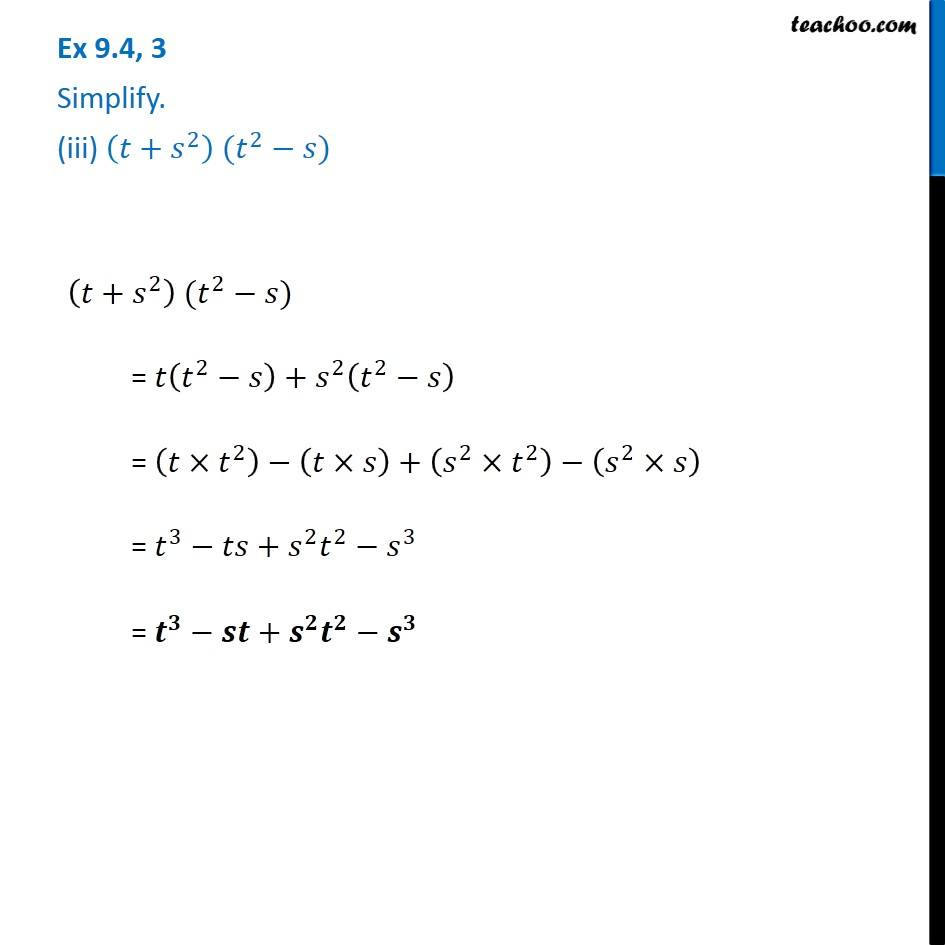Subscribe to our Youtube Channel - https://you.tube/teachoo

1. Chapter 9 Class 8 Algebraic Expressions and Identities
2. Concept wise
3. Multiplication of Polynoimals by Polynomials

Transcript

Ex 9.4, 3 Simplify. (iii) (𝑡+𝑠^2 ) (𝑡^2−𝑠) (𝑡+𝑠^2 ) (𝑡^2−𝑠) = 𝑡(𝑡^2−𝑠)+𝑠^2 (𝑡^2−𝑠) = (𝑡×𝑡^2 )−(𝑡×𝑠)+(𝑠^2×𝑡^2 )−(𝑠^2×𝑠) = 𝑡^3−𝑡𝑠+𝑠^2 𝑡^2−𝑠^3 = 𝒕^𝟑−𝒔𝒕+𝒔^𝟐 𝒕^𝟐−𝒔^𝟑

Multiplication of Polynoimals by Polynomials